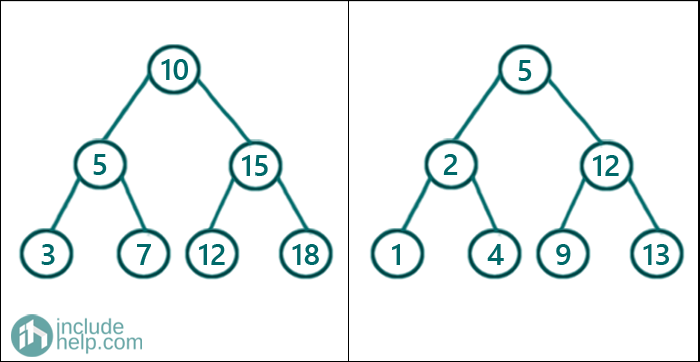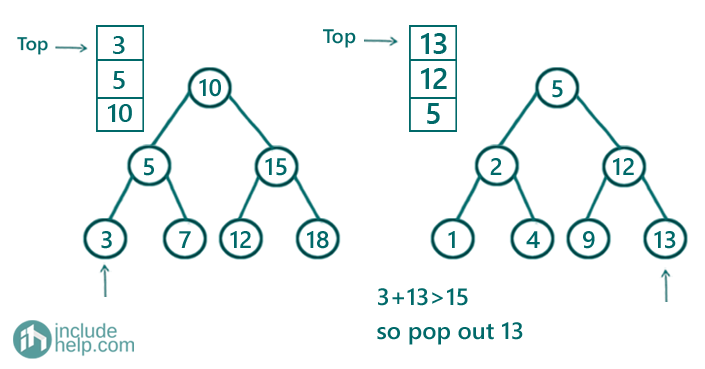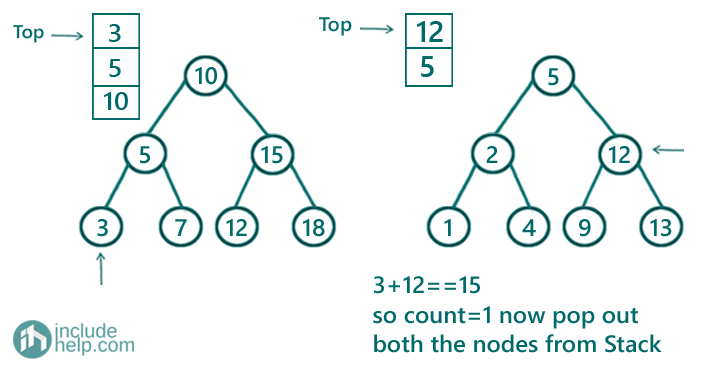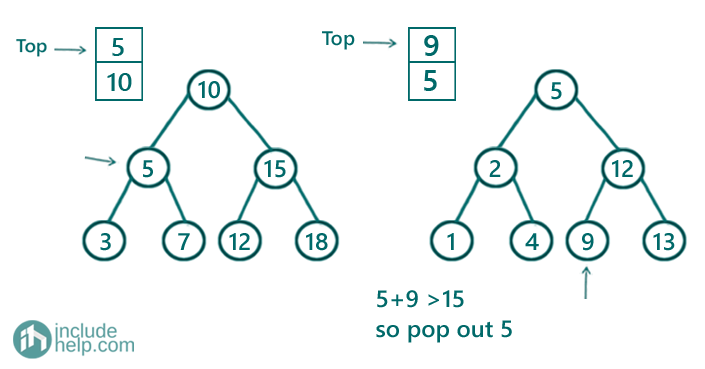# Count Number of pairs from two different BSTs whose sum is equal to X

In this article, we are going to see how to find the number of pairs from two different BSTs whose sum is equal to a given number X?
Submitted by Radib Kar, on November 06, 2020

Prerequisites:

This is similar to our two sum problem where we are given two arrays and we need to find the pairs that will sum to the given sum, X. But in this case instead of two arrays, we have been given two BSTs.

If we think similarly as we solved in the two sum problem, what we need to sort the arrays. We started from the beginning of one array and the end of another array. That was our two-pointer algorithm where we traversed the pointers based on the sum of the current two pointers. For details check the prerequisite article. The reason, we brought it up here is that in case of a BST also, we will use the same two-pointer algorithm.

Say, two of the BSTs are the below ones:If the given sum is 15

Then, we have two pairs

1. [3, 12]: 3 from the first tree & 12 from the second
2. [10, 5]: 10 from the first tree & 5 from the second

Now there is two way to solve the above problem

1. We can store the traversals and convert the BSTs to arrays. In that case, for one BST, we will store the inorder traversal and for another, we will store the reverse inorder traversal. Then we will do the same thing what we did in our past article on the two sum problem.
In this case, thought our time complexity will be O(n) we will have additional space complexity of O(n) and extra stack space for the recursion while doing the inorder and reverse inorder traversal.
2. Another way without storing the traversal, rather implementing the two-pointer algorithm itself while doing the inorder traversal for one Binary Search Tree & reverse inorder traversal for another binary search tree. But of course, here we need iterative traversals to implement the two-pointer algorithm. Before going through the algorithm here please follow the pre-requisite article on iterative inorder traversal.
So, to implement iterative inorder & reverse inorder traversal we need two stacks, namely st1 & st2. Below is the detailed algorithm:
1. Initialize count to 0 which will store the count of pairs
2. while(true){
1. Go to left as long as possible for tree 1 and keep pushing all the nodes into the stack(st1) while going towards left
2. Go to right as long as possible for tree 2 and keep pushing all the nodes into the stack(st2) while going towards left
If either of the stacks is empty
That means we have finished traversal for one of that tree. SO there can't be any more pairs. Thus break the loop.
3. Our current traversing nodes are the top nodes from the stacks. Of course, here we can say, we have two pointers to the nodes.
Since inorder traversal visits the BST in ascending order & reverse inorder traversal visits in descending order, we can do similarly now as we did in our two-pointer algorithm
4. if(sum of node values > x){
That means, we need to decrement pointer from the second tree. So pop out the node and set the next node for traversal.
}
else if sum of node values < x){
That means, we need to increment pointer from the first tree. So pop out the node and set the next node for traversal.
}
else{
that means the sum of two current nodes is equal to x, so increment count and pop out both current nodes from both the stacks and move to next nodes in the traversal.
}
End While

Below is the detailed implementation in C++

```#include <bits/stdc++.h>
using namespace std;

// tree node is defined
class TreeNode {
public:
int val;
TreeNode* left;
TreeNode* right;
TreeNode(int data)
{
val = data;
left = NULL;
right = NULL;
}
};

int countPairs(TreeNode* root1, TreeNode* root2, int x)
{
// Code here
if (root1 == NULL || root2 == NULL)
return 0;

stack<TreeNode *> st1, st2;
int count = 0;
while (true) {
while (root1) {
st1.push(root1);
root1 = root1->left;
}
while (root2) {
st2.push(root2);
root2 = root2->right;
}
if (st1.empty() || st2.empty())
return count;

TreeNode* cur1 = st1.top();
TreeNode* cur2 = st2.top();

if (cur1->val + cur2->val > x) {
st2.pop();
root2 = cur2->left;
}
else if (cur1->val + cur2->val < x) {
st1.pop();
root1 = cur1->right;
}
else {
count++;
st1.pop();
st2.pop();
root1 = cur1->right;
root2 = cur2->left;
}
}
return count;
}

int main()
{
//trees built like the example
TreeNode* root1 = new TreeNode(10);

root1->left = new TreeNode(5);
root1->right = new TreeNode(15);
root1->left->left = new TreeNode(3);
root1->left->right = new TreeNode(7);
root1->right->left = new TreeNode(12);
root1->right->right = new TreeNode(18);

TreeNode* root2 = new TreeNode(5);
root2->left = new TreeNode(2);
root2->right = new TreeNode(12);
root2->left->left = new TreeNode(1);
root2->left->right = new TreeNode(4);
root2->right->left = new TreeNode(9);
root2->right->right = new TreeNode(13);

int sum = 15;

cout << "Number of pairs having sum 15 is: " << countPairs(root1, root2, sum) << endl;

return 0;
}
```

Output:

```Number of pairs having sum 15 is: 2
```

Dry run:

1. We keep going left for the first BST and keep pushing them into the stack1. On the other hand, we keep going right for the second BST and keep pushing them into the stack2. After doing this we have:Now the current nodes would be the top nodes from the stacks which are 3 & 13 respectively and that sum more than 15, thus we will pop out 13 from the stack and set current node for the second tree as 13->left which is NULL here. The current node for the first tree will remain the same as 3.
2. Since 3 has no left child, it won't add anything more to the stack and also will remain as the current node for the first tree. Now current node for the second tree is NULL so that won't add any nodes to stack. So current node for second BST would be current top of its stack which is 12So now we have found a pair and thus we increment count and pop out both current nodes from the stack. Our updated current nodes would be NULL for the first BST(3->right) & 9 for the second BST
3. Since the current node for the first tree is NULL, it doesn't add anything to its stack. But the second tree adds 9 to the stack. So now the stacks are:So now stack tops are 5 & 9 respectively and they sum less than 15, thus we need to pop out 5 and will set current node for tree1 as 7(5->right). Current node for tree2 will remain the same(9).
4. Now, we will add 7 for tree1 stack and tree2 stack will remain same. The top of stacks are now 7 & 9 which sums 16 and that's more than 15. Thus we pop out 9 and set current node for tree 2 as 9->left which is NULL. Current node for tree1 remains the same which is 7
5. Stacks for both the trees are unchanged and current nodes will be 7 and 5. That again sums less than 15 so we pop out 5 from the stack of first BST.
6. Now current nodes are 10 & 5 respectively, which sums to 15, and thus we increment count to 2 and pop out both 10 & 5 while updating current nodes as 15 & 2 respectively.
7. Now we will continue the same way until the stacks become empty for the program to terminate.

So finally count is 2 which is our result.

Top MCQs

Languages: » C » C++ » C++ STL » Java » Data Structure » C#.Net » Android » Kotlin » SQL
Web Technologies: » PHP » Python » JavaScript » CSS » Ajax » Node.js » Web programming/HTML
Solved programs: » C » C++ » DS » Java » C#
Aptitude que. & ans.: » C » C++ » Java » DBMS
Interview que. & ans.: » C » Embedded C » Java » SEO » HR
CS Subjects: » CS Basics » O.S. » Networks » DBMS » Embedded Systems » Cloud Computing
» Machine learning » CS Organizations » Linux » DOS
More: » Articles » Puzzles » News/Updates